## Green's Function

Let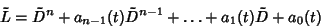(1)

be a differential Operator in 1-D, withContinuous for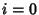, 1, ...,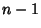on the interval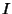, and assume we wish to find the solution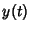to the equation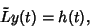(2)

where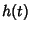is a given Continuous on. To solve equation (2), we look for a function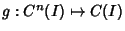such that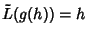, where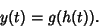(3)

This is a Convolution equation of the form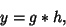(4)

so the solution is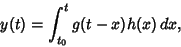(5)

where the function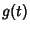is called the Green's function for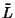on.

Now, note that if we take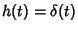, then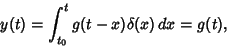(6)

so the Green's function can be defined by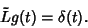(7)

However, the Green's function can be uniquely determined only if some initial or boundary conditions are given.

For an arbitrary linear differential operatorin 3-D, the Green's function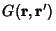is defined by analogy with the 1-D case by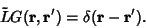(8)

The solution to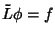is then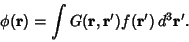(9)

Explicit expressions forcan often be found in terms of a basis of given eigenfunctions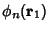by expanding the Green's function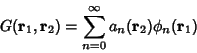(10)

and Delta Function,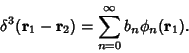(11)

Multiplying both sides by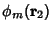and integrating over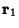space,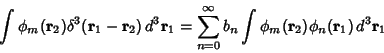(12)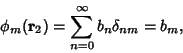(13)

so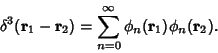(14)

By plugging in the differential operator, solving for the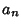s, and substituting into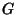, the original nonhomogeneous equation then can be solved.

References

Arfken, G. Nonhomogeneous Equation--Green's Function,'' Green's Functions--One Dimension,'' and Green's Functions--Two and Three Dimensions.'' §8.7 and §16.5-16.6 in Mathematical Methods for Physicists, 3rd ed. Orlando, FL: Academic Press, pp. 480-491 and 897-924, 1985.